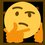# Gaussian Integral

$I\overset { let }{ = } \int _{ -\infty }^{ \infty }{ { e }^{ -{ x }^{ 2 } }dx } =\int _{ -\infty }^{ \infty }{ { e }^{ -{ y }^{ 2 } }dy }$

\begin{aligned} { I }^{ 2 } & = (I)(I) \\ \quad & = (\int _{ -\infty }^{ \infty }{ { e }^{ -{ x }^{ 2 } }dx } )(\int _{ -\infty }^{ \infty }{ { e }^{ -{ y }^{ 2 } }dy } ) \\ \quad & = \int _{ -\infty }^{ \infty }{ \int _{ -\infty }^{ \infty }{ { e }^{ -{ (x }^{ 2 }+{ y }^{ 2 }) }dx } dy } \\ \quad & = \int _{ 0 }^{ 2\pi }{ \int _{ 0 }^{ \infty }{ r{ e }^{ -{ r }^{ 2 } }dr } d\theta } \\ \quad & = \int _{ 0 }^{ 2\pi }{ { \left[ -\frac { { e }^{ -{ r }^{ 2 } } }{ 2 } \right] }_{ 0 }^{ \infty }d\theta } \\ \quad & = \int _{ 0 }^{ 2\pi }{ \frac { 1 }{ 2 } d\theta } \\ \quad & = { \left[ \frac { \theta }{ 2 } \right] }_{ 0 }^{ 2\pi } \\ { I }^{ 2 } & = \pi \\ I & =\sqrt { \pi } \quad (I>0) \end{aligned}

$\boxed { \displaystyle \int _{ -\infty }^{ \infty }{ { e }^{ -{ x }^{ 2 } }dx } =\sqrt { \pi } }$Note by Gandoff Tan
2 years, 1 month ago

This discussion board is a place to discuss our Daily Challenges and the math and science related to those challenges. Explanations are more than just a solution — they should explain the steps and thinking strategies that you used to obtain the solution. Comments should further the discussion of math and science.

When posting on Brilliant:

• Use the emojis to react to an explanation, whether you're congratulating a job well done , or just really confused .
• Ask specific questions about the challenge or the steps in somebody's explanation. Well-posed questions can add a lot to the discussion, but posting "I don't understand!" doesn't help anyone.
• Try to contribute something new to the discussion, whether it is an extension, generalization or other idea related to the challenge.

MarkdownAppears as
*italics* or _italics_ italics
**bold** or __bold__ bold
- bulleted- list
• bulleted
• list
1. numbered2. list
1. numbered
2. list
Note: you must add a full line of space before and after lists for them to show up correctly
paragraph 1paragraph 2

paragraph 1

paragraph 2

[example link](https://brilliant.org)example link
> This is a quote
This is a quote
    # I indented these lines
# 4 spaces, and now they show
# up as a code block.

print "hello world"
# I indented these lines
# 4 spaces, and now they show
# up as a code block.

print "hello world"
MathAppears as
Remember to wrap math in $$ ... $$ or $ ... $ to ensure proper formatting.
2 \times 3 $2 \times 3$
2^{34} $2^{34}$
a_{i-1} $a_{i-1}$
\frac{2}{3} $\frac{2}{3}$
\sqrt{2} $\sqrt{2}$
\sum_{i=1}^3 $\sum_{i=1}^3$
\sin \theta $\sin \theta$
\boxed{123} $\boxed{123}$